# Young’s Double Slits Experiment Derivation

## Introduction To Young’s Double Slits Experiment

During the year 1801 Thomas Young carried out an experiment where the wave and particle nature of light and matter was demonstrated. The schematic diagram of the experimental setup is shown below-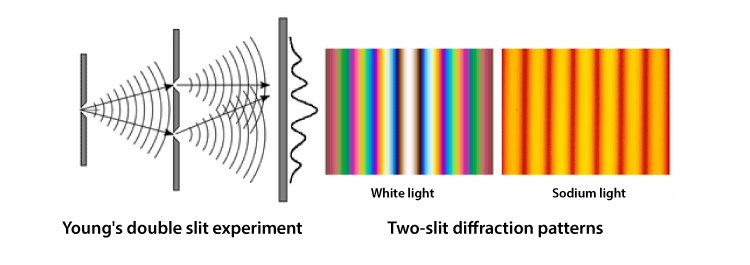Figure(1): Young double slit experimental set up along with fringe pattern.

A beam of monochromatic light is made incident on the first screen, which contains the slit S0. The emerging light then incident on the second screen which consists of two slits namely, S1, S2. These two slits serve as a source of coherent light. The emerging light waves from these slits interfere to produce an interference pattern on the screen. The interference pattern consists of consecutive bright and dark fringes. The dark fringes are the result of destructive interference and bright fringes are the result of constructive interference.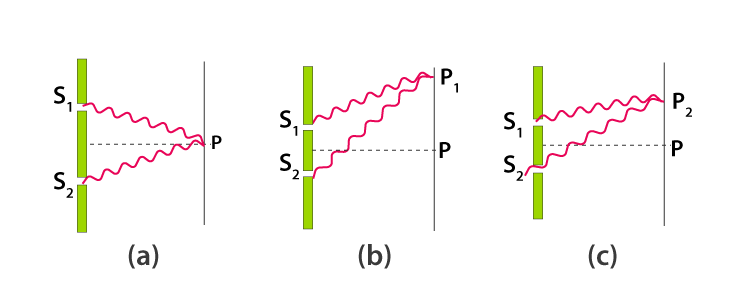Figure(2): shows the interference pattern of two light waves to produce dark or bright fringes. Bright fringe(at P) is formed due to the overlap of two maxima or two minima. Figure 2a,2b . Dark fringe(at P) is formed due to the overlap of maxima with minima. Figure 2c.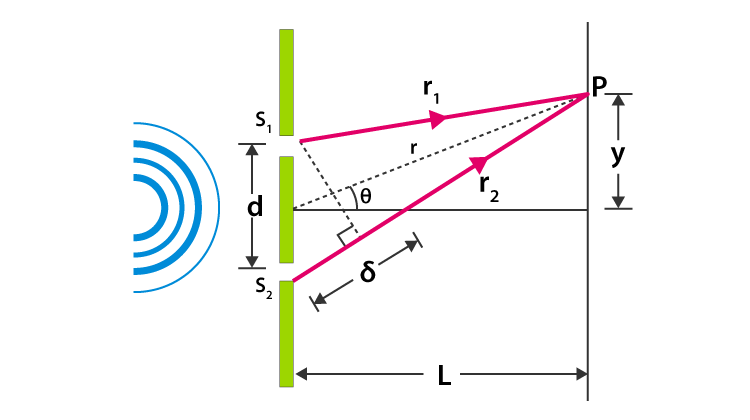Figure(3) Geometry of Young’s double slit interface

The light falls on the screen at the point P. which is at a distance y from the center O.

The distance between the double slit system and the screen is L

The two slits are separated by the distance d

Distance traveled by the light ray from slit 1 to point P on the screen is r1

Distance traveled by the light ray from slit 2 to point P on the screen is r2

Thus, the light ray from slit 2 travels an extra distance of ẟ = r2-r1 than light ray from slit 1.

This extra distance is termed as Path difference.

Refer to Figure(3) Applying laws of cosines; we can write –

$r_{1}^{2} =r^{2}+\left ( \frac{d}{2} \right )^{2}-drcos\left ( \frac{\pi}{2}-\theta \right )$ $=r^{2}+\left ( \frac{d}{2} \right )^{2}-drSin\theta—–(1)$

∵ Cos(90° – θ) = Sinθ

Similarly, $r_{2}^{2} =r^{2}+\left ( \frac{d}{2} \right )^{2}-drcos\left ( \frac{\pi}{2}+\theta \right )$ $=r^{2}+\left ( \frac{d}{2} \right )^{2}+drSin\theta—–(2)$

∵ Cos(90° + θ) = -Sinθ

Subtracting equation (2) from (1) we get-

$r_{2}^{2}-r_{1}^{2}=\left ( r^{2}+\left ( \frac{d}{2} \right )^{2}+drSin\theta \right )-\left ( r^{2}+\left ( \frac{d}{2} \right )^{2}-drSin\theta \right )$ $=2drSin\theta —–(3)$

Let us impose the limit that the distance between the double slit system and the screen is much greater than the distance between the slits. That is L >> d. The sum of r1 and r2 can be approximated to r1 + r2 ≅2r. Thus, the path difference becomes –

$\delta =r_{2}-r_{1}\approx dSin\theta—–(4)$

In this limit, the two rays r1 and r2 are essentially treated as parallel. (See Figure(4))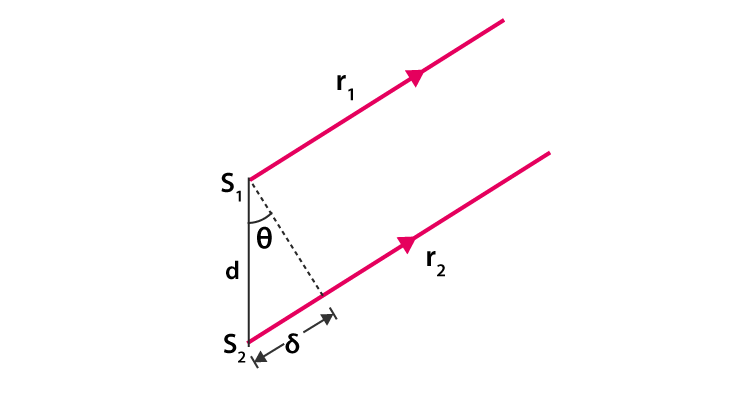Figure(4): Assuming L >> d, The path difference between two rays.

To compare the phase of two waves, the value of path difference (ẟ) plays a crucial role.

Constructive interference is seen when path difference (ẟ) is zero or integral multiple of the wavelength(λ).

 (Constructive interference) ẟ = dSin𝜃 = mλ —-(5), m = 0, ±⁤1, ±2, ±3, ±4, ±5, ……

Where m is order number. The Zeroth order maximum (m=0 )corresponds to the central bright fringe, here 𝜃=0. The first order maxima(m=±⁤1)(bright fringe) are on either side the central fringe.

Similarly, when ẟ is an odd integral multiple of λ/2, the resultant fringes will be 1800 out of phase, thus, forming dark fringe.

 (Distructive interference) ẟ = dSin𝜃 = $\left [ m+\frac{1}{2} \right ]$λ—(6), m = 0, ±⁤1, ±2, ±3, ±4, ±5, ……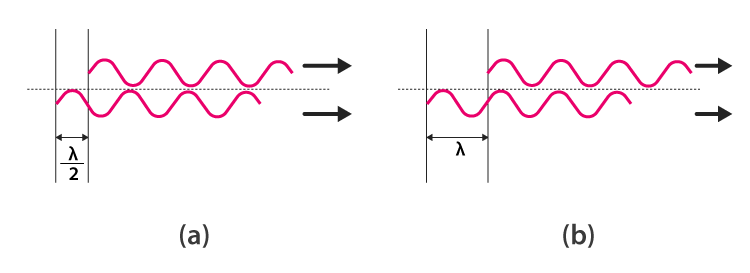Figure(5)(a) How path difference ẟ = λ/2 (m=0) results in distructive interference. (b) ẟ = λ (m=1) yeilds constructive interence.

Assuming the distance between the slits are much greater than the wavelength of the incident light, we get-

$sin\theta \approx tan\theta=\frac{y}{L}$

Substituting it in the constructive and destructive interference condition we can get the position of bright and dark fringes, respectively. The equation is as follow-

 $y_{b}=m\frac{\lambda L}{d}$

And

 $y_{d}=\left [ m+\frac{1}{2} \right ]\frac{\lambda L}{d}$

Physics related links:

Stay tuned with Byju’s for more interesting articles.

#### Practise This Question

The angle of incidence for an equilateral prism is 60. What should be the refractive index of prism so that the ray is parallel to the base inside the prism?